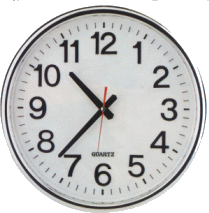Posted on

# Clock

Clocks

How long is a day?
It's the amount of time it takes for the Earth to rotate one time on its axis. But how long does it take the Earth to rotate? That is where things become completely arbitrary. The world has decided to standardize on the following increments:A day consists of two 12-hour periods, for a total of 24 hours.

1. An hour consists of 60 minutes using a thick short hand.
2. A minute consists of 60 seconds using a thick long hsnd.
3. Seconds are subdivided on a decimal system into things like "hundredths of a second" or "millionths of a second" using a thin long hand, usually a different color.

That's a pretty bizarre way to divide a day up. We divide it in half, then divide the halves by twelfths, then divide the twelfths into sixtieths, then divide by 60 again, and then convert to a decimal system for the smallest increments. It's no wonder children have trouble learning how to tell time!